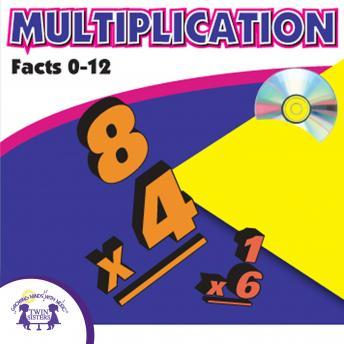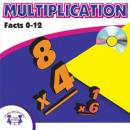# Rap With The Facts - MULTIPLICATION

#### Summary:

Students can learn, drill and review the facts of 12 in only 8-minutes. This is a fun and interesting way for your children to learn the basics of multiplication! Children can sing along and these songs will be stuck in their head before you know it!
Track List:
2. Facts of 2 (Multiplication - WITH ANSWERS)
3. Facts of 3 (Multiplication - WITH ANSWERS)
4. Facts of 4 (Multiplication - WITH ANSWERS)
5. Facts of 5 (Multiplication - WITH ANSWERS)
6. Facts of 6 (Multiplication - WITH ANSWERS)
7. Facts of 7 (Multiplication - WITH ANSWERS)
8. Facts of 8 (Multiplication - WITH ANSWERS)
9. Facts of 9 (Multiplication - WITH ANSWERS)
10. Facts of 10 (Multiplication - WITH ANSWERS)
11. Facts of 11 (Multiplication - WITH ANSWERS)
12. Facts of 12 (Multiplication - WITH ANSWERS)
14. Facts of 2 (Multiplication - WITHOUT ANSWERS)
15. Facts of 3 (Multiplication - WITHOUT ANSWERS)
16. Facts of 4 (Multiplication - WITHOUT ANSWERS)
17. Facts of 5 (Multiplication - WITHOUT ANSWERS)
18. Facts of 6 (Multiplication - WITHOUT ANSWERS)
19. Facts of 7 (Multiplication - WITHOUT ANSWERS)
20. Facts of 8 (Multiplication - WITHOUT ANSWERS)
21. Facts of 9 (Multiplication - WITHOUT ANSWERS)
22. Facts of 10 (Multiplication - WITHOUT ANSWERS)
23. Facts of 11 (Multiplication - WITHOUT ANSWERS)
24. Facts of 12 (Multiplication - WITHOUT ANSWERS)
Runtime: 16:02:00

## Rap With The Facts - MULTIPLICATION

#### by Twin Sisters Productions##### Enter your email below to be notified as soon as it is available!

By clicking "Notify Me" you consent to receiving electronic marketing communications from Audiobooks.com. You will be able to unsubscribe at any time.